# Correlation is a Cosine

You might have heard a statement of the kind “correlation is a cosine” but you have not bothered enough to investigate what it means precisely. It certainly sounds interesting. How can the simplest bivariate summary statistic be related to a trigonometric function from sixth grade? What is the relation between correlation and cosines?

Let me explain.

As with anything else, a Google search is your friend here, with many links to helpful Stack Overflow posts explaining this connection from all sorts of angles. However, I do find John D. Cook’s blog post most helpful, and I am following his exposition closely.

##### Piece #1: The Law of Cosines

The law of cosines states that in any triangle with sides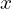,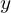, and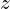and an angle (betweenand)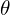, we have:

(1)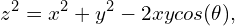In the special case when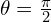, the term on the right-hand side equals 0 and the equation reduces to the well-known Pythagorean Theorem.

##### Piece #2: The Variance of the Sum of Two Random Variables

Let’s imagine two random variables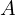,. The variance of their sum is given by: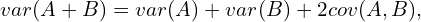where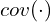, denotes covariance. We can substitute the last term with its definition as follows: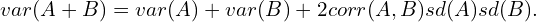Next, we know that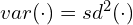. Substituting, we get:

(2)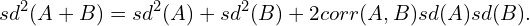##### Piece #3: Putting the Two Equations Together

Setting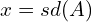,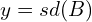, and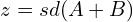in equation (1) gives the desired result. With one small caveat – the negative sign on the cosine term. To get around this we can simply look at the complementary angle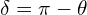.

That is, we imagine a triangle with sides equal to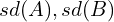and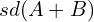, whereis the angle between. When this angle is small (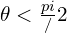), the two sides point in the same direction andandare positively correlated. The opposite is true for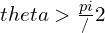. As mentioned above,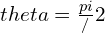kills the correlation term, consistent withandbeing independent.

##### Summary

In short, the formula for the variance of two random variables follows the law of cosines. Substituting and arranging terms shows the desired result.